# OpenCV-Python 高动态范围（HDR）

• 了解如何从曝光序列生成和显示 HDR 图像。
• 使用曝光融合来合并曝光序列。

## 曝光顺序 HDR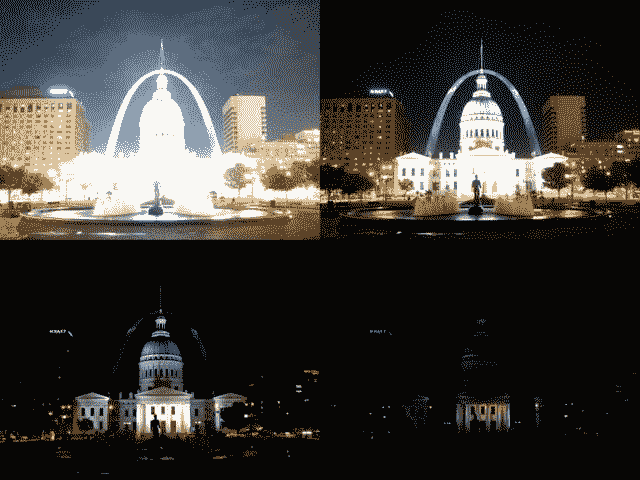### 1.将曝光图像加载到列表中

``````import cv2 as cv
import numpy as np

img_fn = ["img0.jpg", "img1.jpg", "img2.jpg", "img3.jpg"]
img_list = [cv.imread(fn) for fn in img_fn]
exposure_times = np.array([15.0, 2.5, 0.25, 0.0333], dtype=np.float32)
``````

### 2.将曝光合并到 HDR 图像中

``````# Merge exposures to HDR
imagemerge_debevec = cv.createMergeDebevec()
hdr_debevec = merge_debevec.process(img_list, times=exposure_times.copy())
merge_robertson = cv.createMergeRobertson()
hdr_robertson = merge_robertson.process(img_list, times=exposure_times.copy())
``````

### 3. Tonemap HDR 图像

``````# Tonemap HDR image
tonemap1 = cv.createTonemapDurand(gamma=2.2)
res_debevec = tonemap1.process(hdr_debevec.copy())
tonemap2 = cv.createTonemapDurand(gamma=1.3)
res_robertson = tonemap2.process(hdr_robertson.copy())
``````

### 4.使用 Mertens 融合合并曝光

``````# Exposure fusion using Mertens
merge_mertens = cv.createMergeMertens()
res_mertens = merge_mertens.process(img_list)
``````

### 5.转换为 8 位并保存

``````# Convert datatype to 8-bit and save
res_debevec_8bit = np.clip(res_debevec*255, 0, 255).astype('uint8')
res_robertson_8bit = np.clip(res_robertson*255, 0, 255).astype('uint8')
res_mertens_8bit = np.clip(res_mertens*255, 0, 255).astype('uint8')

cv.imwrite("ldr_debevec.jpg", res_debevec_8bit)
cv.imwrite("ldr_robertson.jpg", res_robertson_8bit)
cv.imwrite("fusion_mertens.jpg", res_mertens_8bit)
``````

## 结果

### 德普外阁(Debevec)：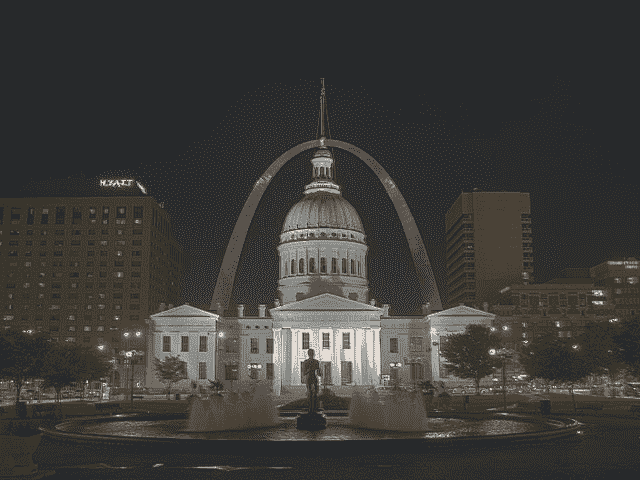### 罗伯逊(Robertson)：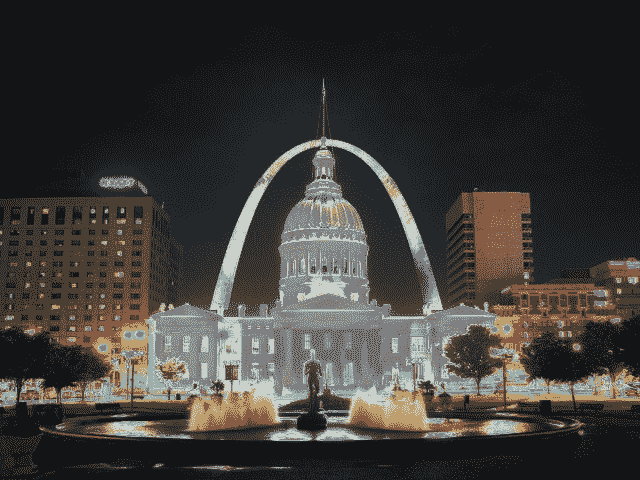### 梅特内斯·福森(Mertenes Fusion)：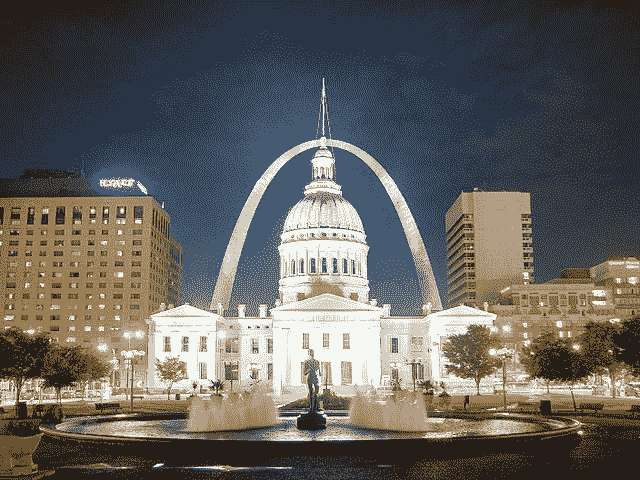## 估计相机响应功能

``````# Estimate camera response function (CRF)
cal_debevec = cv.createCalibrateDebevec()
crf_debevec = cal_debevec.process(img_list, times=exposure_times)

hdr_debevec = merge_debevec.process(img_list,   times=exposure_times.copy(),response=crf_debevec.copy())

cal_robertson = cv.createCalibrateRobertson()
crf_robertson = cal_robertson.process(img_list, times=exposure_times)

hdr_robertson = merge_robertson.process(img_list, times=exposure_times.copy(), response=crf_robertson.copy())
``````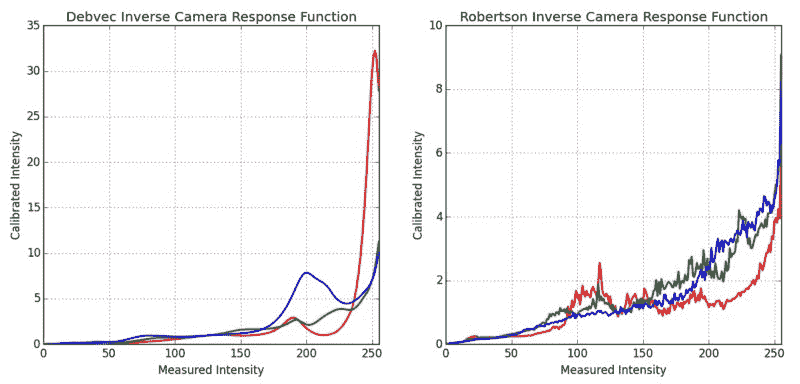## 其他资源

1. Paul E Debevec 和 Jitendra Malik。从照片中恢复高动态范围辐射图。在 ACM SIGGRAPH 2008 课程中，第 31 页.ACM，2008。
2. Mark A Robertson，Sean Borman 和 Robert L Stevenson。通过多次曝光改善动态范围。在 Image Processing，1999。ICIP 99.会议录。 1999 年国际会议，第 3 卷，第 159-163 页。 IEEE，1999。
3. Tom Mertens，Jan Kautz 和 Frank Van Reeth。曝光融合。在计算机图形和应用程序，2007 年.PG'07。第 15 届太平洋会议，第 382-390 页。 IEEE，2007。
4. 来自 Wikipedia-HDR 的图片

• 回顶